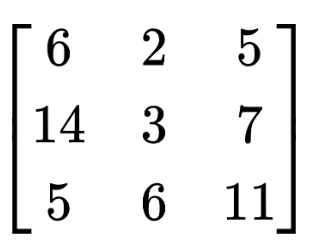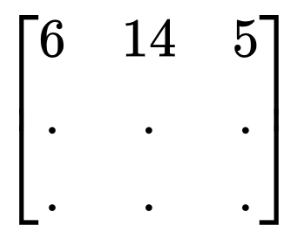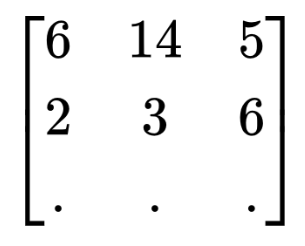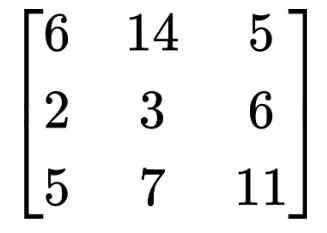# Transpose Matrix Calculator

To find the transpose of a matrix, select the order of the matrix, Input the entries of the matrix, and click calculate button using matrix transpose calculator## Matrix Transpose Calculator

Find the transpose of any matrix using this calculator. This matrix transpose calculator will find the transpose of the matrix of order from 2x2 to 5x5.

## What is the transpose of a matrix?

Transpose in an operation that is applied to the matrices. It changes the arrangement of rows and columns. Rows become columns and vice versa.

It is denoted by a little “t” at the corner of the variable representing the matrix e.g At. Interestingly enough, the diagonal of the matrix does not change.

## How to find the transpose of a matrix?

The main point to remember when taking the transpose of a matrix is to be careful with the order of the rows and columns.

The first column becomes the first row and the second column becomes the second row. If there are more columns, the same rule is applied to them.

Example:

Find the transpose of the following matrix.Solution:

Step 1: Make an empty 3 by 3 matrix. Write the first column as row number 1.Step 2: After that, take the entries of the second column and write at the place of the second row.Step 3: Do the same for the third column.This is the transpose of the given matrix.